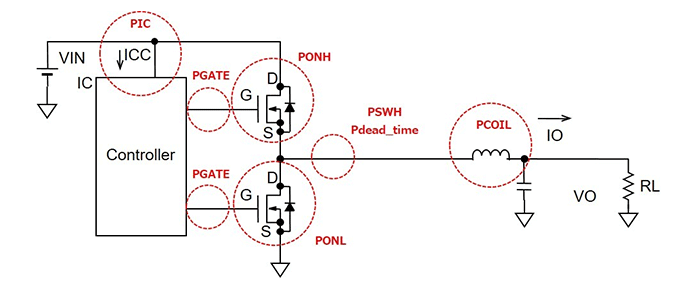# Losses in Synchronous Rectifying Step-Down Converters

2018.08.09

・The loss in a synchronous rectifying step-down converter is the sum of the losses of the various components.

From here, we embark on a study of losses in synchronous rectifying step-down converters. We begin by identifying the places at which losses occur in a synchronous rectifying step-down converter. Then, we consider the losses at each point.

## Sites of Losses in a Synchronous Rectifying Step-Down Converter

Below is shown a simplified circuit of a synchronous rectifying step-down converter, indicating places at which losses occur. Loss occurrence sites are indicated by abbreviated names, in red.PONH is the conduction loss due to the ON-resistance when the high-side MOSFET is turned on.

PONL is the conduction loss due to the ON-resistance when the low-side MOSFET is turned on.

PSWH is the switching loss of the MOSFETs.

Pdead_time is the dead time loss. When the timing is such that there is an interval in which the high-side MOSFET and low-side MOSFET are both turned on simultaneously, VIN and GND are nearly in a short-circuited state, and an overcurrent, sometimes called a shoot-through current or the like, flows. In order to avoid this, nearly all controller ICs cause both the high-side and the low-side MOSFETs to be turned off for a very short interval when switching the two MOSFETs on and off. This is called the dead time. It is necessary to ensure safety, but results in a loss.

PIC is the power supply current for a power supply IC, in this case, the controller IC for the synchronous rectifying step-down converter with external power transistors. In essence, this is the current consumed by the IC itself, and is the self current consumption.

PGATE is the gate charge loss of the external MOSFET. As a rule, current does not flow in a MOSFET gate, but the charge to drive the gate capacitance is necessary, and this becomes a loss. Losses occur in both the high-side and the low-side MOSFETs.

PCOIL is the conduction loss due to the DC resistance (DCR) of the output inductor.

The result of adding all of these losses is the loss of the synchronous rectifying step-down converter.

PONH：Conduction losses due to ON-resistance of the high-side MOSFET while on
PONL：Conduction losses due to ON-resistance of the low-side MOSFET while on
PSWH：Switching losses
PIC：IC self current consumption loss
PGATE：Gate charge losses
PCOIL： Conduction losses due to the inductor DCR

From the next section, we will study individual losses.